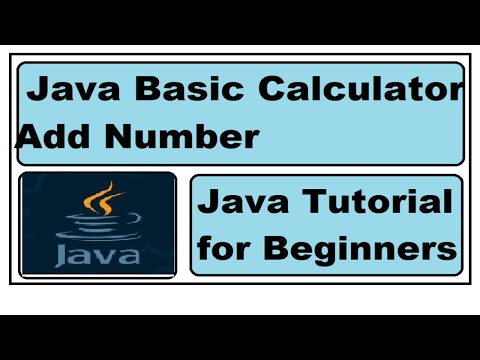## Wednesday, November 30, 2022

### How to learn variable Data type Program | Java Tutorial for Beginners

In this tutorial we will learn about how to create variable to java data type and how to use it with practical step by step example.

If you want to follow along, click on video tutorial which are available in Hindi and English languages.

Java  primitive Data type tutorial in English

Java  primitive Data type tutorial in Hindi

Java Integer

If we want to use or declare  integer, we type int then name of variable in this example point is name of variable which hold value which 5 and for assign  we use =.  since we store value in variable call point now we can use it. lets print out  in console.  we have main method  and print in console we have system.out.println command and we use semicolon at the end .Now click on Green button to run program but first save before run and check in console. we can observe 5 in console. Integer uses 4 bytes of memory and can store whole number start form -2147483648 to 2147483647.

Java Primitive data type Byte
Byte is  primitive datatype of Java. which can hold whole number from -128 to 127 and use 1 byte of size.
to declare byte we type byte and created variable(num) which hold value 10 and we will print it . Now save program and click on run and observe you can 10 in console as describe in image.

Short is another datatype of Java which can hold whole number from -32768to 32767 and use size of 2 bytes.  To declare short we use short and created variable name price which hold value 15. for assign we use equal sign and we are printing value in console as we can observe in below picture.
Long is one of the data type of Java and we can use if we have large number , since it can hold large number as result it will take 8 bytes of memory and can hold whole number from -9223372036854775808 to 9223372036854775807. To declare long we use long as data type , we use store as name of variable and printing in console.
Java Primitive data type Float
All above data type we use for if number is whole but if number is in fraction or not whole number? In Java we use float or double so lets see how to use it.
Float can hold 6 to 7 fractional number and take 4 bytes of memory .
where double can hold 15 decimal number and take 8 bytes of  memory. To declare double datatype we use double and we assign value 3.25 to variable name cake and we are printing this in main method . we can see 3.25 value is print out in out put.
If we want to store single character we can use this data type in Java. Which can store single value and take 2 byte of memory .  Lets take an example. To declare character we use char as data type and we used  math as variable for value we use single invited comma as described in below image.
If we want to use more that one character we can use String . Unlike character we will provide double invited comma to value . lets take and example of String. To declare string we use string as datatype and we assign value in last name variable now we will print and check out put.
Boolean is either true or false . This is very important data type  we will use this in future tutorial a lot. Boolean will take 1 byte of  memory.  lets check an example. To declare Boolean data type we use boolean and we created variable and set as true and we printed in console

Now here important things to know we can use same data type for many circumstances but if we know how much memory it uses we can use or choose data type wisely for an example if we want to store value as 25 .  we can use byte , shorts, int, long but is it good idea ? check memory usage and select what data type is ideal to use. If you would like to lean more please click on below link

## Structured Self-Development (SSD) Level 2 Module 1, 2, and 3

### Assignment of Arithmetic Operators Java | Tutorial for Beginners

In this tutorial you will learn how to create and call non static method . We will create method and do operation of addition, subtraction...